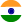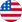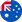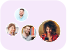24*7 support on WhatsAppChat NowProduct Edition:2nd Edition
Author: Ramin S. Esfandiari
Book Name: Numerical Methods for Engineers and Scientists Using MATLABÃ‚Â®
Subject Name: Maths

# Numerical Methods for Engineers and Scientists Using MATLABÃ‚Â® 2nd Edition Solutions

0 out of 5.0
26 reviews29 Students
have requested for homework help from this book

This book provides a pragmatic, methodical and easy-to-follow presentation of numerical methods and their effective implementation using MATLAB, which is introduced at the outset. The author introduces techniques for solving equations of a single variable and systems of equations, followed by curve fitting and interpolation of data. The book also provides detailed coverage of numerical differentiation and integration, as well as numerical solutions of initial-value and boundary-value problems. The author then presents the numerical solution of the matrix eigenvalue problem, which entails approximation of a few or all eigenvalues of a matrix. The last chapter is devoted to numerical solutions of partial differential equations that arise in engineering and science. Each method is accompanied by at least one fully worked-out example showing essential details involved in preliminary hand calculations, as well as computations in MATLAB.

5
13
4
5
3
1
2
8
1
0

0

## Students who viewed this book also checked out